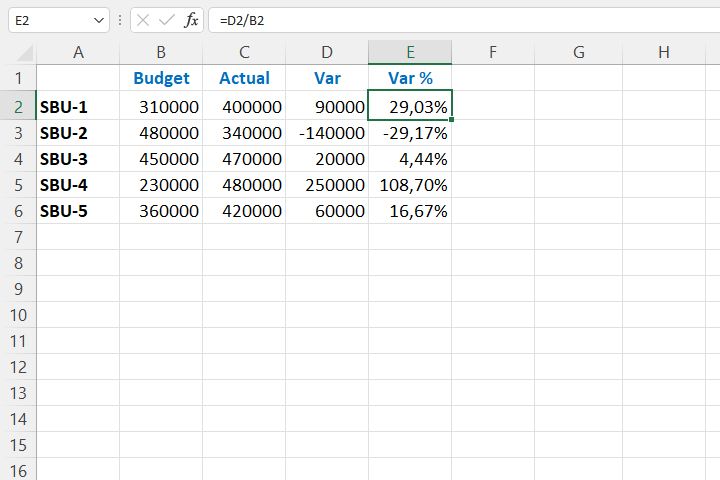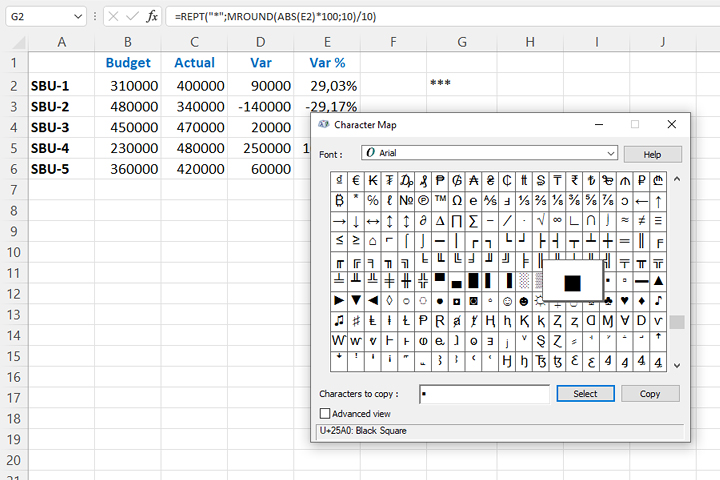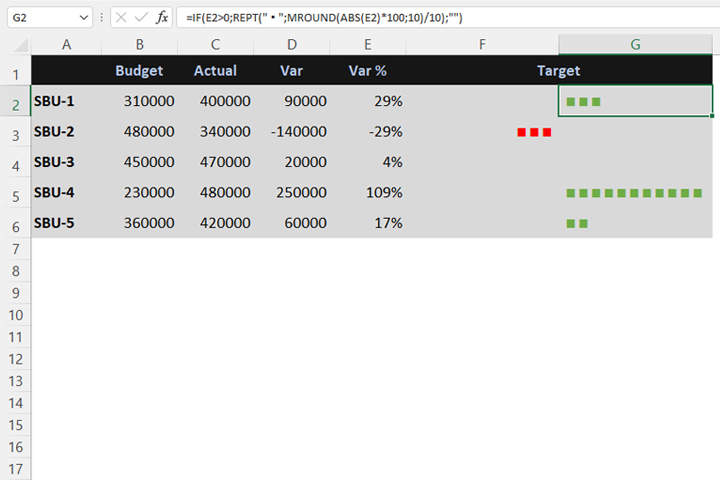# LED chart

The LED chart is not a chart, in the true sense of the word, but it looks nice and is a good choice to illustrate Variance analysis report. We make it so that, after analyzing the percentage for which the plan was exceeded or missed, with the help of the REPT function, generate a certain number of “squares” to illustrate the obtained result.

We will start with the table in which we analyze Variance. The first two columns show the Budget and Actual values. We analyze the results by strategic business units, although here it could be a product, brand or some other criterion for which we want to perform an analysis.

Variance analysis can be done in several ways, it all depends on how you want to see the results. Analysts often subtract ACTUAL from the BUDGET column because they do not want to get a negative Variance. It seems to me that it is a more correct way than to subtract the BUDGET  from the ACTUAL, because in this way we see whether we have exceeded or failed the plan. So, in column D we subtract the planning from the realized values:

=C2-B2

while in column E we divide the values ​​from column D by those from column B to calculate the percentage.

=D2/B2We will create a graphical representation of the value using the REPT function, which allows the symbols to be repeated the desired number of times. If the variance is negative, the result will be displayed in the left column. Otherwise, it will be displayed in the right column. Let’s say each symbol represents 10% of the value. How to write a formula that repeats a given number of times? In cell G2 we should enter the expression:

=MROUND(ABS(E2)*100,10)/10

The ABS function calculates the absolute value of the percentage of variance, and MROUND rounds the expression to tenths. Let’s include this expression in the symbol repetition function.

=REPT(“*”,MROUND(ABS(E2)*100,10)/10)

Then, using the Character Map Windows application, you need to find the symbol for the “square”. You can choose others, be creative. Replace “star” with the selected symbol.We will include this expression in the IF function which examines whether the value is greater than or less than zero. In column G we will create the formula:

=IF(E2>0,REPT(“▪”,MROUND(ABS(E2)*100,10)/10),””)

and in column F:

=IF(E2<0,REPT(“▪”,MROUND(ABS(E2)*100,10)/10),””)

We will align the values ​​in column F to the right, and those in column G to the left. A little more formatting and you will get an effective report.